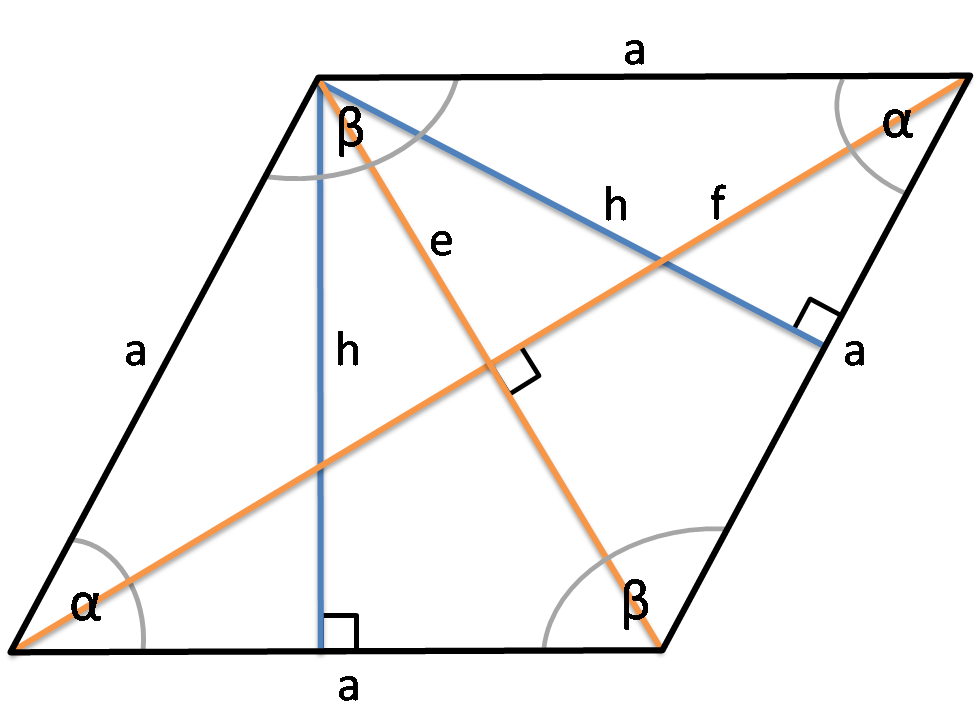# Rhombus Area Calculator

Created by Hanna Pamuła, PhD candidate
Reviewed by Bogna Szyk
Last updated: Mar 11, 2022

Rhombus area calculator is a great tool to determine the area of a rhombus, as well as its perimeter and other characteristics: diagonals, angles, side length, and height. Have a look on many ways you can find the diamond area: given diagonals of a rhombus, using base and height, side and any chosen angle... Are you still wondering how to find the area of rhombus or rhombus perimeter? Check the rhombus area formulas below or just experiment with the tool.

## Rhombus and its properties

A rhombus is a simple quadrilateral with all sides equal. The other names are an equilateral quadrilateral or a diamond (like the one from playing cards ♢).Fundamental properties of a rhombus are:

• The two diagonals of a rhombus are perpendicular and bisect each other,
• Its diagonals bisect opposite angles,
• Opposite angles have equal measure.

Every rhombus is a parallelogram and a kite.

## Rhombus area formula

There are three useful formulas for calculation of the area of the rhombus:

1. Knowing base and height

area = base * height

1. Knowing diagonals of a rhombus

area = (e * f)/2

1. Knowing side and any (!) angle

area = s² * sin(angle)

Why can we use any angle in the last rhombus area formula? Because we know that two adjacent angles are supplementary, and sin(angle) = sin(180° - angle).

There are other variations of those equations (as e.g. calculating the area given height and angle), but they are only simple trigonometric transformations of those three most popular rhombus area formulas.

## Rhombus perimeter

Finding the rhombus perimeter is trivial if we know the side length - it's 4 * a. But what if we know only diagonals of a rhombus? Let's check:

1. We know that diagonals are perpendicular and bisect each other. So the rhombus is nothing else than four congruent triangles, with legs equal to e/2 and f/2.
2. All we need to do is finding the hypotenuse of the triangle. You can use here the right triangle calculator or Pythagorean theorem calculator.
3. Multiply by 4 the obtained hypotenuse value. It's your rhombus perimeter!

Also, you can use this formula:

• perimeter = 4 * √(e/2)²-(f/2)²)

Or just type the lengths of the diagonals into the rhombus area calculator!

## How to find the area of a rhombus?

Are you still pretty unsure how to use the calculator? Let's show its potential on the simple example:

1. Type the first given value you have. Let's assume it's side = 10 in.
2. Type the second given value. For example, an angle equal to 30°.
3. Wow! The rhombus area calculator is displaying all the other values - area, height, perimeter, angle and diagonals. Impressive, isn't it?

Our tool is really flexible - if it's possible to calculate, it will do it. Usually two given values are enough, give it a try!

## Is square a rhombus? Or is a rhombus a parallelogram?

The answer is yes to both questions. Every square is a rhombus, as for a rhombus the only necessary condition is that it needs to have all sides of equal length. As you know perfectly well, a square needs to have all sides equal and all four equal angles, so it fulfills the conditions to be a rhombus.

Similarly, a rhombus is a parallelogram, as any shape needs to have two pairs of parallel sides to be a parallelogram - and the rhombus has them. So the rhombus is always a parallelogram, but parallelogram is a rhombus only in a special case - for a parallelogram with four sides of equal length.

Hanna Pamuła, PhD candidateSide (a)
in
Height (h)
in
Shorter diagonal (f)
in
Longer diagonal (e)
in
Angle α
deg
Angle β
deg
Area & Perimeter
Area
in²
Perimeter
in
People also viewed…

### Car crash force

With this car crash calculator, you can find out how dangerous are car crashes.

### Ellipse standard form

In our ellipse standard form calculator, you can determine the standard form of an ellipse by inputting its vertices.

### Snowman

The perfect snowman calculator uses math & science rules to help you design the snowman of your dreams!

### Triangle ratio

The triangle ratio calculator allows you to determine the angles in a triangle when you know their ratio.